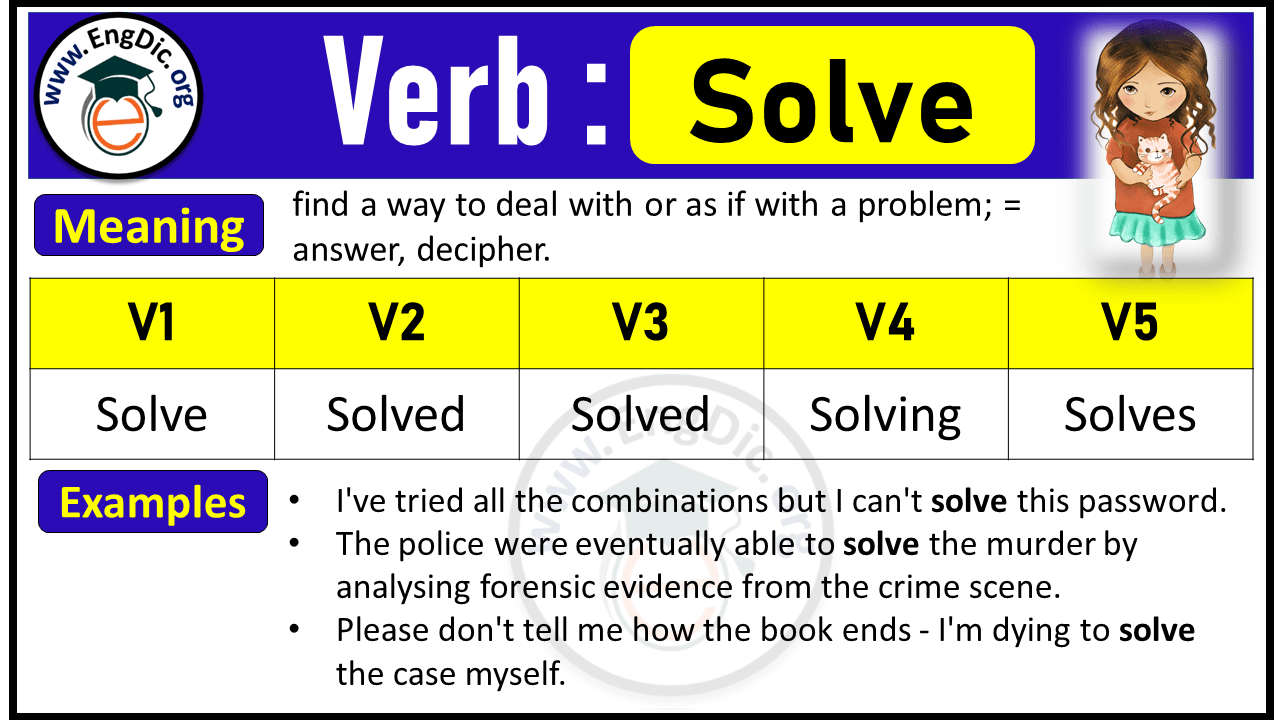# Solve Verb Forms: Past Tense and Past Participle (V1 V2 V3)

Meaning: find a way to deal with or as if with a problem

## Solve Verb Forms V1 V2 V3 V4 V5

 Infinitive/Base Form (V1): Solve Past Tense (V2): Solved Past Participle Form (V3): Solved Present Participle/Gerund (V4): Solving 3rd Person Singular (V5): Solves

## Solve Past Tense:

Past Tense of Solve is Solved.

Example: Sarah solved the equation.

## Solve Past Participle:

Past Participle Form of Solve is Solved.

Example: Sarah has solved the equation.

## Solve Present Participle:

Present Participle Form of Solve is Solving.

Example: Sarah is solving the equation.

## Solve 3rd Person Singular:

3rd Person Singular of Solve is Solves.

Example: Sarah solves the equation.## Solve Conjugation

### Indefinite / Simple Present Tense

• I solve the equation.
• We/You/They solve the equation.

### Present Continuous Tense

• I am solving the equation.
• We/You/They are solving the equation.
• He/She/It/Adam is solving the equation.

### Present Perfect Tense

• I have solved the equation.
• We/You/They have solved the equation.
• He/She/It/Adam has solved the equation.

### Present Perfect Continuous Tense

• I have been solving the equation.
• We/You/They have been solving the equation.
• He/She/It/Adam has been solving the equation.

### Indefinite / Simple Past Tense

• I solved the equation.
• We/You/They solved the equation.

### Past Continuous Tense

• I was solving the equation.
• We/You/They were solving the equation.
• He/She/It/Adam was solving the equation.

### Past Perfect Tense

• I had solved the equation.
• We/You/They had solved the equation.

### Past Perfect Continuous Tense

• I had been solving the equation.
• We/You/They had been solving the equation.

### Indefinite / Simple Future Tense

• I will solve the equation.
• We/You/They will solve the equation.
• He/She/It/Adam will solve the equation.

### Future Continuous Tense

• I will be solving the equation.
• We/You/They will be solving the equation.
• He/She/It/Adam will be solving the equation.

### Future Perfect Tense

• I will have solved the equation.
• We/You/They will have solved the equation.
• He/She/It/Adam will have solved the equation.

### Future Perfect Continuous Tense

• I will have been solving the equation.
• We/You/They will have been solving the equation.
• He/She/It/Adam will have been solving the equation.

## Past Tense of Solve Phrasal Verbs

 Solve Phrasal Verbs Past Tense Solve for Solved for Solve out Solved out Solve up Solved up Solve over Solved over Solve with Solved with Solve in Solved in Solve about Solved about Solve on Solved on Solve through Solved through Solve down Solved down

## FAQS

### What is the Future Tense of Solve?

Future Tense of solve is “will solve”.

### What is the Present Tense of Solve?

Present Tense of solve is “solve + s/es or ing”.

 I/we/you/they solve He/she/it/singular name solves Present participle solving

### What is the Past Perfect Tense of Solve?

Past perfect tense of take is “had solved”.

Explore Other Verb Forms:

Last updated on May 22nd, 2023 at 04:16 am